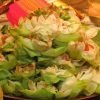How Cheenta works to ensure student success?
Explore the Back-Story

# Circular arc | AMC 10A ,2012 | Problem No 18Try this beautiful Problem on Geometry from Circular arc from (AMC 10 A, 2012).

## Circular arc - AMC-10A, 2012- Problem 18

The closed curve in the figure is made up of 9 congruent circular arcs each of length $\frac{2 \pi}{3},$ where each of the centers of the corresponding circles is among the vertices of a regular hexagon of side $2 .$ What is the area enclosed by the curve?

,

• $2 \pi+6$
• $2 \pi+4 \sqrt{3}$
• $3 \pi+4$
• $2 \pi+3 \sqrt{3}+2$
• $\pi+6 \sqrt{3}$

Geometry

Circle

Hexagon

## Suggested Book | Source | Answer

Pre College Mathematics

AMC-10A, 2012

#### Check the answer here, but try the problem first

$\pi+6 \sqrt{3}$

## Try with Hints

#### First Hint

We have to find out the area enclosed by the curve. but we can not find out eassily. Now we join the centre of 9 congruent circles. it will form a hexagon.

Can you find out the required area of the closed curve? Can you finish the problem?

#### Second Hint

The area of the Hexagon = $(6)(0.5)(2 \sqrt{3})=6 \sqrt{3}$. But we have to find out the area area enclosed by the curve. Then add the areas of the three sectors outside the hexaqon and subtract the areas of the three sectors inside the hexagon but outside the fiqure to get the area enclosed in the curved figure

#### Third Hint

The areas of the three sectors outside the hexaqon=$2 \pi$ .

the areas of the three sectors inside the hexagon but outside the figure $(\pi)$

Therefore the area enclosed by the curve is $\pi+6 \sqrt{3}$

## Subscribe to Cheenta at Youtube

Try this beautiful Problem on Geometry from Circular arc from (AMC 10 A, 2012).

## Circular arc - AMC-10A, 2012- Problem 18

The closed curve in the figure is made up of 9 congruent circular arcs each of length $\frac{2 \pi}{3},$ where each of the centers of the corresponding circles is among the vertices of a regular hexagon of side $2 .$ What is the area enclosed by the curve?

,

• $2 \pi+6$
• $2 \pi+4 \sqrt{3}$
• $3 \pi+4$
• $2 \pi+3 \sqrt{3}+2$
• $\pi+6 \sqrt{3}$

Geometry

Circle

Hexagon

## Suggested Book | Source | Answer

Pre College Mathematics

AMC-10A, 2012

#### Check the answer here, but try the problem first

$\pi+6 \sqrt{3}$

## Try with Hints

#### First Hint

We have to find out the area enclosed by the curve. but we can not find out eassily. Now we join the centre of 9 congruent circles. it will form a hexagon.

Can you find out the required area of the closed curve? Can you finish the problem?

#### Second Hint

The area of the Hexagon = $(6)(0.5)(2 \sqrt{3})=6 \sqrt{3}$. But we have to find out the area area enclosed by the curve. Then add the areas of the three sectors outside the hexaqon and subtract the areas of the three sectors inside the hexagon but outside the fiqure to get the area enclosed in the curved figure

#### Third Hint

The areas of the three sectors outside the hexaqon=$2 \pi$ .

the areas of the three sectors inside the hexagon but outside the figure $(\pi)$

Therefore the area enclosed by the curve is $\pi+6 \sqrt{3}$

## Subscribe to Cheenta at Youtube

This site uses Akismet to reduce spam. Learn how your comment data is processed.

### 5 comments on “Circular arc | AMC 10A ,2012 | Problem No 18”

1.DEB JYOTI MITRA says:

I have A Big DOUBT:
In Problem:It should be 6 congruent circular arcs, instead of 9 congruent circular arcs,
The closed curve in the figure is made up of 6 congruent circular arcs each of length 2π/3, where each of the centers of the corresponding circles is among the vertices of a regular hexagon of side 2. What is the area enclosed by the curve?
Pl Check If Problem needs Correction OR AM I Wrong?

1.DEB JYOTI MITRA says:

I realised the problem is All-Right.
It is made of circular sectors of circle of radius of 1;
Three Outside sectors Of Hexagon: each of reflex angles (4/3)*π;
Three Inside Sectors Of Hexagon each of obtuse angle (2/3)* π;
Area of HeHexagon =6X (1/2)*2*√3=6*√3;
Three ouside sectors are equivalent to two circle of radiuus 1; To be added in area of hexagon
AND Three inside sectors are equivalent to one circle of radius 1; to be substracted in the area of hexagon;
Area of Figure provided= 6*√3+π* (1)^2=6*√3+1

1.DEB JYOTI MITRA says:

Area of Figure provided= 6*√3+π* (1)^2=6*√3+1 is to be read as
6*√3+π

2.KOUSHIK SOM says:

i think it will be 9 congruent

1.DEB JYOTI MITRA says:

Yes,I realised it is nine Congruent ones!

### Knowledge Partner